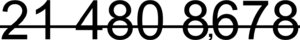Numerical digit facts for kids

Kids Encyclopedia FactsThese three numbers are not three digits; they are numbers made of digits. (e.g. "21" has two digits just as "480" has three digits.)

Numerical digits are the number text characters used to show numerals. For example, the numeral "56" has two digits: 5 and 6. In the decimal system (which is base 10), each digit is how many of a certain power of 10 are needed to get the value. The rightmost, or units digit, is for 10^0, the next digit is for 10^1, etc.

The numeral "56" means:  6*10^0 + 5*10^1 = 6*1 + 5*10 = 6 + 50.

The ten digits of the decimal system are: 0, 1, 2, 3, 4, 5, 6, 7, 8, and 9. Some numeral systems need more than ten digits. For example, the hexadecimal numeral system uses 16 digits: 0, 1, 2, 3, 4, 5, 6, 7, 8, 9, A, B, C, D, E, and F.

Related pagesNumerical digit Facts for Kids. Kiddle Encyclopedia.